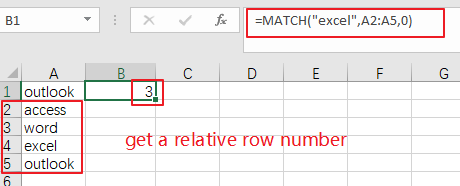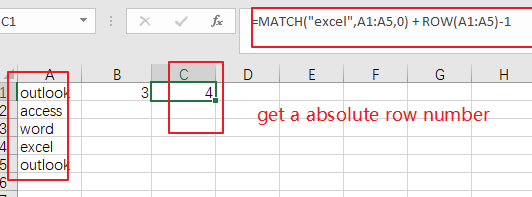# How to Get Row Number From a Vlookup in Excel

This post will guide you how to get row number from a Vlookup in Excel. How do I return a row number from a Vlookup with a formula in Excel.

Assuming that you have a list of data in range A1:B5, you can use the VLOOKUP function to lookup a value in the first column and then return the corresponding cell value in the same row. But now I do not want to get the cell value, and I want to get the row number from a VLookup in Excel. How to accomplish it.

## Get Row Number From a Vlookup

If you want to return a row number from a Vlookup, you can use a formula based on the MATCH function. For example, you want to lookup a string value “excel” in range A1:A5 and return the row number where the searching value is found. Like this:

`=MATCH("excel",A2:A5,0)`

You need to type this formula into a desired cell and press Enter key to apply this formula. You would see that the row number returned.Note: the above formula only returns a relative row number, and if you want to get a absolute row number, you can use another formula based on the ROW function and the MATCH function, like this:

`=MATCH("excel",A1:A5,0) + ROW(A1:A5)-1`

Type this formula into a blank cell and press Enter key to apply it.### Related Functions

• Excel VLOOKUP function
The Excel VLOOKUP function lookup a value in the first column of the table and return the value in the same row based on index_num position.The syntax of the VLOOKUP function is as below:= VLOOKUP (lookup_value, table_array, column_index_num,[range_lookup])….
• Excel ROW function
The Excel ROW function returns the row number of a cell reference.The ROW function is a build-in function in Microsoft Excel and it is categorized as a Lookup and Reference Function.The syntax of the ROW function is as below:= ROW ([reference])….
• Excel MATCH function
The Excel MATCH function search a value in an array and returns the position of that item.The syntax of the MATCH function is as below:= MATCH  (lookup_value, lookup_array, [match_type])….

Sidebar